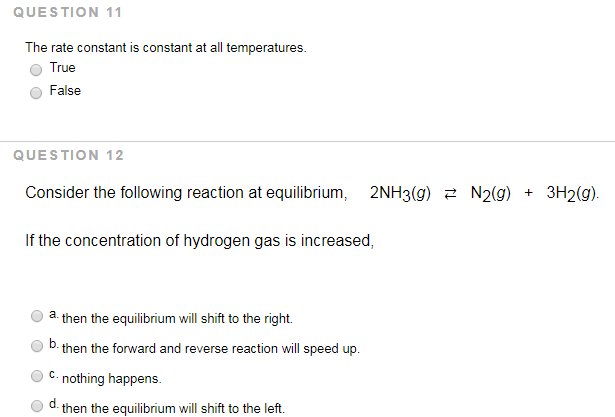# Problem: QUESTION 11The rate constant is constant at all temperatures.            a. True            b. False QUESTION 12Consider the following reaction at equilibrium, 2NH3 (g) ⇌ N2 (g) + 3H2 (g). If the concentration of hydrogen gas is increased,           a. then the equilibrium will shift to the right.           b. then the forward and reverse reaction will speed up.           c. nothing happens.           d. then the equilibrium will shift to the left.

###### FREE Expert Solution
94% (346 ratings)###### Problem Details

QUESTION 11

The rate constant is constant at all temperatures.

a. True

b. False

QUESTION 12

Consider the following reaction at equilibrium, 2NH3 (g) ⇌ N2 (g) + 3H2 (g).

If the concentration of hydrogen gas is increased,

a. then the equilibrium will shift to the right.

b. then the forward and reverse reaction will speed up.

c. nothing happens.

d. then the equilibrium will shift to the left.